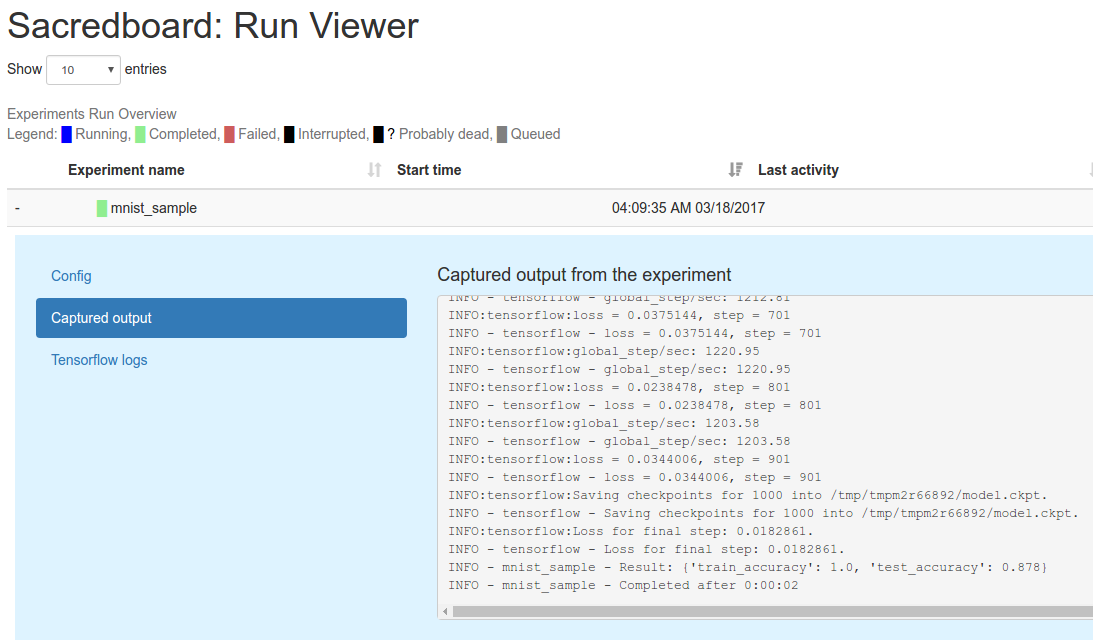# 用 Sacred 追蹤實驗數據

## 前言

「大大都怎麼跑實驗阿？」

「包成 Docker 先放著。或是用 Makefile + GitLab 做版本管控。 用 Jenkins + ZooKeeper 管理，然後隨時打點 OpenTSDB 用 Grafana 監控。」

## 環境設定

``pip3 install sacred==0.7b3 pymongo``

``pip3 install xgboost jupyter matplotlib numpy tensorflow-gpu scikit-learn sacredboard``

## 主程式

``````# mnist.py
from sacred import Experiment
ex = Experiment('mnist_sampled')

@ex.config
def my_config():
sample_size = 500
classifier = 'svc'
``````

``````# mnist.py
from sklearn.metrics import accuracy_score
from tensorflow.examples.tutorials.mnist import input_data

@ex.automain
def run_experiments(data_dir='MNIST_data'):
X_test = mnist.test.images
y_test = mnist.test.labels

X_train, y_train = sample_data(mnist.train.images, mnist.train.labels)

clf = get_classifier()
clf.fit(X_train, y_train)
y_train_pred = clf.predict(X_train)
train_accuracy = accuracy_score(y_train, y_train_pred)

y_test_pred = clf.predict(X_test)
test_accuracy = accuracy_score(y_test, y_test_pred)

return {'train_accuracy': train_accuracy, 'test_accuracy': test_accuracy}
``````

``````# mnist.py
import numpy as np
import xgboost as xgb

from sklearn.svm import LinearSVC

from softmax import SoftmaxClassifier

@ex.capture
def sample_data(X, y, sample_size, _rnd):
indices = np.arange(X.shape)
choice_indices = _rnd.choice(indices, sample_size)
return X[choice_indices, :], y[choice_indices]

@ex.capture
def get_classifier(classifier):
if classifier == 'svc':
return LinearSVC()
elif classifier == 'softmax':
return SoftmaxClassifier()
elif classifier == 'xgb':
return xgb.XGBClassifier()
else:
return None
``````

SoftmaxClassifier 的程式我放在 softmax.py。 如有興趣可自行參考。

## 執行實驗

``````#!/bin/bash

for clf in svc xgb softmax ;
do
for sample_size in 500 1000 1500 ;
do
for seed in 1 2 3 4 5 6 7 8 9 10 ;
do
python mnist.py with classifier=\$clf sample_size=\$sample_size seed=\$seed -m sacred || exit 1
done
done
done
``````

## 回收結果

``sacredboard -m sacred````````from pymongo import MongoClient
mc = MongoClient()
db = mc['sacred']
``````

``````import numpy as np

sample_sizes = (500, 1000, 1500)
classifiers = ('svc', 'xgb', 'softmax')

results = []
for clf in classifiers:
clf_mean = []
clf_std = []
for sample_size in sample_sizes:
test_accuracy = []
for seed in range(1, 11):
result = db['runs'].find_one({'experiment.name': 'mnist_sampled',
'config.classifier': clf,
'config.sample_size': sample_size,
'config.seed': seed,
'status': 'COMPLETED'},
{'result': 1})
test_accuracy.append(result['result']['test_accuracy'])
clf_mean.append(np.mean(test_accuracy))
clf_std.append(np.std(test_accuracy))
results.append((clf_mean, clf_std))
``````

``````import matplotlib.pyplot as plt

fig, ax = plt.subplots(nrows=1, ncols=1, figsize=(8, 6))

ax.set_title('Test Accuracy of Different Classifiers on MNIST')
ax.set_xlabel('sample size')
ax.set_ylabel('test accuracy')

xticks = np.arange(len(sample_sizes))
ax.set_xticks(xticks)
ax.set_xticklabels(sample_sizes)

for i, clf in enumerate(classifiers):
ax.errorbar(xticks, results[i], yerr=results[i], label=clf)
ax.legend(bbox_to_anchor=(1, -0.1))

plt.show()
``````

Yong-Siang Shih